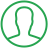# Fibonacci Series In Java Using While Loop

Last Updated : Jul 1, 2023In this article we will show you the solution of Fibonacci series in java using while loop, set the initial and subsequent second numbers as 0 and 1, respectively. We then publish the initial and second numbers after that.

The flow is then transferred to an iterative while loop, where the two previous numbers are added to obtain the next number, and the first and second numbers are simultaneously swapped with the third.

The compiler has been included so that you can run the set of programmes yourself, including appropriate examples and sample outputs.

We will examine the many approaches to find the Fibonacci Series In Java Program for the first n numbers.

After creating an object of the "Fibonacci" class, call the method using the object as "f.fib(num)", after which fib(int n) starts the execution with n=5 and calls itself with n=n-1, before starting the execution of the function once more.

This process continues until the condition "if(n>0)" is false.

Throughout the Fibonacci sequence, the one term inside is equal to the sum of the two before it.

A Fibonacci sequence begins with zero and ends with one.

0,1,2,3,4, 8,12,21,and 34 in the Fibonacci sequence

The sum of the two digits before the next digit in the Fibonacci sequence, such example, 0,1,2,3,4,8,12,21,34, and 55. The first 2 digits in the Fibonacci series are zero and one.

The Fibonacci series programme can be written in Java in two different ways:

• Using the Fibonacci Series without recursion
• Recursive Fibonacci Series

## Step By Step Guide On Fibonacci Series In Java Using While Loop :-

```public class TalkersCodeExample {
public static void main(String[] args) {
int count = 10, num1 = 0, num2 = 1;
System.out.print("Fibonacci Series of "+count+" numbers:");
int i=1;
while(i<=count)
{
System.out.print(num1+" ");
int sumOfPrevTwo = num1 + num2;
num1 = num2;
num2 = sumOfPrevTwo;
i++;
}
}
}```
1. First we create a public class with the name of TalkersCodeExample.
2. Then we create a public static void main as string args.
3. After that we count an integer number with num1, and num2.
4. After that we create the Fibonacci Series of "+count+" numbers using system.out.println.
5. After that we define the while loop as a count.
6. After that we define previous numbers using system.out.println.

## Conclusion :-

A number in the Fibonacci numbers is calculated by adding the two preceding integers. The series goes 0,1,2,3,4,8,12,21,34, and etc, beginning with 0 and 1.

We'll create three scripts to print the Fibonacci sequence here. using the for loop 2) implementing a while loop 3) according to the entered number.

I hope this article on Fibonacci series in java using while loop helps you and the steps and method mentioned above are easy to follow and implement.

##About Anjali

Experienced Computer Programmer with a broad range of experience in technology. Strengths in application development and Object Oriented architecture design, front end programming, usability and multimedia technology. Expert in coding languages such as C, C++ Java, JavaScript, PHP and more.